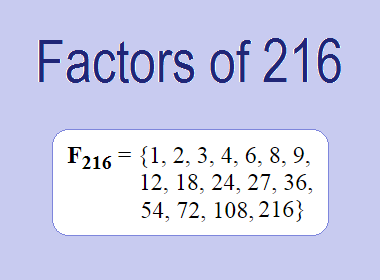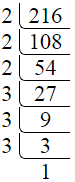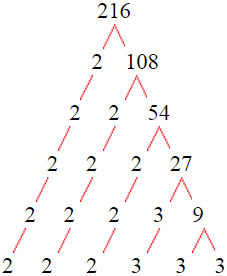# Factors of 216The factors of 216 are 1, 2, 3, 4, 6, 8, 9, 12, 18, 24, 27, 36, 54, 72, 108, and 216 i.e. F216 = {1, 2, 3, 4, 6, 8, 9, 12, 18, 24, 27, 36, 54, 72, 108, 216}. The factors of 216 are all the numbers that can divide 216 without leaving a remainder.

We can check if these numbers are factors of 216 by dividing 216 by each of them. If the result is a whole number, then the number is a factor of 216. Let's do this for each of the numbers listed above:

·        1 is a factor of 216 because 216 divided by 1 is 216.

·        2 is a factor of 216 because 216 divided by 2 is 108.

·        3 is a factor of 216 because 216 divided by 3 is 72.

·        4 is a factor of 216 because 216 divided by 4 is 54.

·        6 is a factor of 216 because 216 divided by 6 is 36.

·        8 is a factor of 216 because 216 divided by 8 is 27.

·        9 is a factor of 216 because 216 divided by 9 is 24.

·        12 is a factor of 216 because 216 divided by 12 is 18.

·        18 is a factor of 216 because 216 divided by 18 is 12.

·        24 is a factor of 216 because 216 divided by 24 is 9.

·        27 is a factor of 216 because 216 divided by 27 is 8.

·        36 is a factor of 216 because 216 divided by 36 is 6.

·        54 is a factor of 216 because 216 divided by 54 is 4.

·        72 is a factor of 216 because 216 divided by 72 is 3.

·        108 is a factor of 216 because 216 divided by 108 is 2.

·        216 is a factor of 216 because 216 divided by 216 is 1.

## How to Find Factors of 216?

1 and the number itself are the factors of every number. So, 1 and 216 are two factors of 216. To find the other factors of 216, we can start by dividing 216 by the numbers between 1 and 216. If we divide 216 by 2, we get a remainder of 0. Therefore, 2 is a factor of 216. If we divide 216 by 3, we get a remainder of 0. Therefore, 3 is also a factor of 216.

Next, we can check if 4 is a factor of 216. If we divide 216 by 4, we get a remainder of 0. Therefore, 4 is also a factor of 216. If we divide 216 by 5, we get a remainder of 1. Therefore, 5 is not a factor of 216. We can continue this process for all the possible factors of 216.

Through this process, we can find that the factors of 216 are 1, 2, 3, 4, 6, 8, 9, 12, 18, 24, 27, 36, 54, 72, 108, and 216. These are the only numbers that can divide 216 without leaving a remainder.

********************

********************

## Properties of the Factors of 216

The factors of 216 have some interesting properties. One of the properties is that the sum of the factors of 216 is equal to 600. We can see this by adding all the factors of 216 together:

1 + 2 + 3 + 4 + 6 + 8 + 9 + 12 + 18 + 24 + 27 + 36 + 54 + 72 + 108 + 216 = 600

Another property of the factors of 216 is that the prime factors of 216 are 2, and 3 only.

## Applications of the Factors of 216

The factors of 216 have several applications in mathematics. One of the applications is in finding the highest common factor (HCF) of two or more numbers. The HCF is the largest factor that two or more numbers have in common. For example, to find the HCF of 216 and 120, we need to find the factors of both numbers and identify the largest factor they have in common. The factors of 216 are 1, 2, 3, 4, 6, 8, 9, 12, 18, 24, 27, 36, 54, 72, 108, and 216. The factors of 120 are 1, 2, 3, 5, 6, 8, 10, 12, 15, 20, 24, 30, 40, 60, and 120. The largest factor that they have in common is 24. Therefore, the HCF of 216 and 120 is 24.

Another application of the factors of 216 is in prime factorization. Prime factorization is the process of expressing a number as the product of its prime factors. The prime factors of 216 are 2, and 3 since these are the only prime numbers that can divide 216 without leaving a remainder. Therefore, we can express 216 as:

216 = 2 × 2 × 2 × 3 × 3 × 3

We can do prime factorization by division and factor tree method also. Here is the prime factorization of 216 by division method,216 = 2 × 2 × 2 × 3 × 3 × 3

Here is the prime factorization of 216 by the factor tree method,216 = 2 × 2 × 2 × 3 × 3 × 3

## Conclusion

The factors of 216 are the numbers that can divide 216 without leaving a remainder. The factors of 216 are 1, 2, 3, 4, 6, 8, 9, 12, 18, 24, 27, 36, 54, 72, 108, and 216. The factors of 216 have some interesting properties, such as having a sum of 600. The factors of 216 have several applications in mathematics, such as finding the highest common factor and prime factorization.